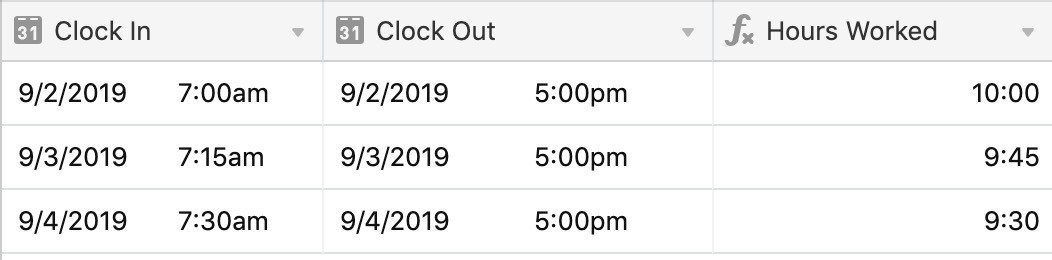• English
• Account
• API
• Integrations
• Enterprise
• Resources
Calculating the difference between dates in Airtable
• 04 Dec 2023
• Dark
Light
• PDF

# Calculating the difference between dates in Airtable

• Dark
Light
• PDF

Article Summary

This article covers how to calculate the difference between two dates in Airtable.

## Introduction

 Plan availability All plan types Platform(s) Web/Browser, Mac app, and Windows app Related reading

NOTE
• "Payment Received" and "Invoice Billed" are the exact names of our two date fields in the example below. You'll need to apply the examples below to the fields in your particular Airtable instance.
• If your base contains date strings, in a single line text field for example, then you will need to use the DATETIME_PARSE() formula function to convert the dates from strings into a DateTime format that Airtable can use in any subsequent `DATETIME_DIFF()` formulas.

## Understanding the DATETIME_DIFF() function with examples

Using the DATETIME_DIFF() function in the example below, we'll find the difference - in days - between two dates.

``````DATETIME_DIFF(
{Invoice Billed},
'days'
)
``````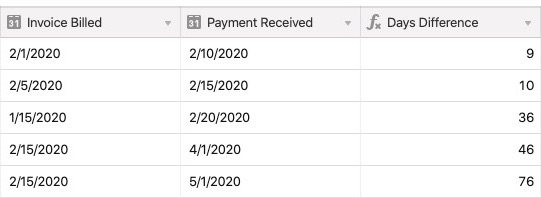Another common use of `DATETIME_DIFF()` is to find the difference between a record's date and today's date. You can use `DATETIME_DIFF()` in combination with the `TODAY()` formula to dynamically find the difference from today's date.

``````DATETIME_DIFF(
TODAY(),
{Invoice Billed},
'days'
)
``````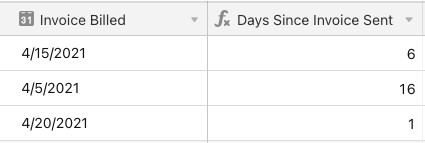For another example, you can also use `DATETIME_DIFF` to find someone's age when given their birthday:

``````DATETIME_DIFF(
{Birth Date},
NOW(),
'years'
) * -1
``````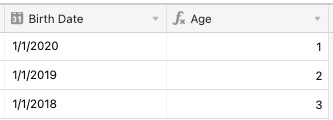## Displaying in decimal format

In another example, if you need to measure the time an employee spends working each day, you could create two date fields to track their clock-in and clock-out times. Using a DATETIME_DIFF() function like the following, you can display the total hours worked for the day.

``````DATETIME_DIFF({Clock Out},{Clock In},'minutes')  / 60
``````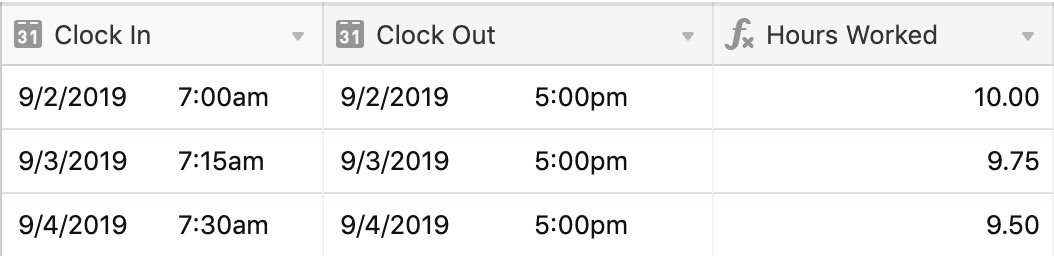## Displaying in duration format

Since duration fields store values in seconds, you can tweak the formula to output the DATETIME_DIFF() value in seconds instead of minutes.  That way, if we format it as a duration using h:mm, we get the expected result.

``````DATETIME_DIFF({Clock Out},{Clock In},'seconds')
``````